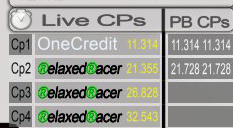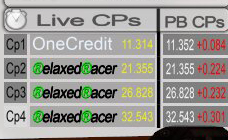Page 3 of 4

### Re: [Plugin]cpDiff (Checkpoint-Time-Differences)

Posted: 04 Aug 2018, 15:29
aca wrote:
04 Aug 2018, 13:32
Hi RelaxedRacer,

nice, that you like the plugin. The fact, that the finish is shown also as cp was intended, as like this you can (if you're slower than a hunted dedi for example) see in one place, how much slower you were and also how much time you lost in the last sector.

At the moment I don't spend time in the game and also not at programming, I'm afraid your request must wait for a while. When I'm back in game and have some spare time for programming, I'll have a look at your wish again - although I don't quite understand, why showing the finish should be bad.

Greetz, aca
Ok i will wait for that thanx!
I want this becouse i want this widget and the best cp's widget side by side, but than the finish time sticks out.
I understand it is intended, but i realy would like to only show the cp'sMaybe nice idea have it optional and to be able to move the "Fin: time" somewhere els, just a thought.
Thanx again, greets ®elaxed®acer

### Re: [Plugin]cpDiff (Checkpoint-Time-Differences)

Posted: 30 Jan 2019, 18:49
Sorry for the long time I was not present here. If you still want the adapted plugin, RelaxedRacer, here we go:

If I understand correctly, you only want to show the CPs (not the finish) on top and you don't use middle-widget or bottom-widget of my plugin. Is this the case, you just need to replace the code between lines 1042 and 1063 in plugin.checkpoint_time_differences.php

Code: Select all

``````		//TimeDiffWidget top
\$posXtop = (int)\$this->settings['WIDGET_TOP']['POS_X'];
\$posYtop = (int)\$this->settings['WIDGET_TOP']['POS_Y'];
\$cpNoColor = \$this->settings['WIDGET_TOP']['CPNO_COLOR'];
\$cpTimeColorTop = \$this->settings['WIDGET_TOP']['CP_TIME_COLOR'];

\$column = 0;
\$cp = 0;
while(\$cp < \$this->checkpointCount){
\$xml .= '<frame pos="'. (\$posXtop + 22 * \$column).' '.\$posYtop.'" z-index="0" id="FrameCheckpointTimeDiffTop'.\$cp.'">';
\$xml .= '<label pos="2 0" z-index="0.02" size="8 3.75" textsize="2" scale="0.8" text="' .((\$cp + 1 == \$this->checkpointCount)? "Fin: " : "Cp".(\$cp + 1).": ").'" valign="center" textcolor="'.\$cpNoColor.'" />';
\$xml .= '<label pos="10 0" z-index="0.02" size="10 3.75" textsize="2" textcolor="'.\$cpTimeColorTop.'" text="" scale="0.8" valign="center" id="LabelTopCheckpoint'.\$cp.'"/>';
\$xml .= '</frame>';

\$column++;
if(\$column == \$numCols){
\$posYtop -= 6;
\$column = 0;
}
\$cp++;
}
``````
with the following one:

Code: Select all

``````		//TimeDiffWidget top
\$posXtop = (int)\$this->settings['WIDGET_TOP']['POS_X'];
\$posYtop = (int)\$this->settings['WIDGET_TOP']['POS_Y'];
\$cpNoColor = \$this->settings['WIDGET_TOP']['CPNO_COLOR'];
\$cpTimeColorTop = \$this->settings['WIDGET_TOP']['CP_TIME_COLOR'];

\$column = 0;
\$cp = 0;
while(\$cp < \$this->checkpointCount){
\$xml .= '<frame pos="'. (\$posXtop + 22 * \$column).' '.\$posYtop.'" z-index="0" id="FrameCheckpointTimeDiffTop'.\$cp.'">';
\$xml .= '<quad pos="0 0" z-index="0.01" size="20 5" valign="center" id="QuadTopCheckpoint'.\$cp.'" hidden="'.((\$cp + 1 == \$this->checkpointCount)? "true" : "false").'"/>';
\$xml .= '<label pos="2 0" z-index="0.02" size="8 3.75" textsize="2" scale="0.8" text="' .((\$cp + 1 == \$this->checkpointCount)? "Fin: " : "Cp".(\$cp + 1).": ").'" valign="center" textcolor="'.\$cpNoColor.'" hidden="'.((\$cp + 1 == \$this->checkpointCount)? "true" : "false").'"/>';
\$xml .= '<label pos="10 0" z-index="0.02" size="10 3.75" textsize="2" textcolor="'.\$cpTimeColorTop.'" text="" scale="0.8" valign="center" id="LabelTopCheckpoint'.\$cp.'" hidden="'.((\$cp + 1 == \$this->checkpointCount)? "true" : "false").'"/>';
\$xml .= '</frame>';

\$column++;
if(\$column == \$numCols){
\$posYtop -= 6;
\$column = 0;
}
\$cp++;
}
``````
then the finish won't be shown anymore.

If this is not the wanted solution, don't hesitate to write back and I'll do my best to make it fitGreetz, aca

### Re: [Plugin]cpDiff (Checkpoint-Time-Differences)

Posted: 01 Feb 2019, 21:02
aca wrote:
30 Jan 2019, 18:49
If this is not the wanted solution, don't hesitate to write back and I'll do my best to make it fitGreetz, aca
Thanx man, this is exacly what i wanted, tested it, works perfect!

One requestis it posible to also display the checkpoint times so its like:

cp1 0:01.234 +0.123
cp2 0:12.345 -0.678
etc

Thanx again### Re: [Plugin]cpDiff (Checkpoint-Time-Differences)

Posted: 03 Feb 2019, 13:40
No problem, you're welcome.
RelaxedRacer wrote:
01 Feb 2019, 21:02
One requestis it posible to also display the checkpoint times so its like:

cp1 0:01.234 +0.123
cp2 0:12.345 -0.678
etc
This is possible, I'm working on it (will not take half a year this time)

### Re: [Plugin]cpDiff (Checkpoint-Time-Differences)

Posted: 03 Feb 2019, 21:23
aca wrote:
03 Feb 2019, 13:40

This is possible, I'm working on it (will not take half a year this time)thanx!

### Re: [Plugin]cpDiff (Checkpoint-Time-Differences)

Posted: 05 Feb 2019, 16:23For adding the cp-time, you need to change:

1. line 939

Code: Select all

``````CpTimeDiffLabelsTop[Int].Value = TimeToTextDiff(CpTime) ^" "^ Tcolor ^ TimeToTextDiff(MathLib::Abs(TimeDiff));
``````
2. line 1018

Code: Select all

``````CpTimeDiffLabelsTop[CurrentCheckpoint -1].Value = TimeToTextDiff(PlayersCurrentCheckpoints[PlayerPlayingLogin][CurrentCheckpoint -1]) ^" "^ TextColor ^ TimeToTextDiff(MathLib::Abs(TimeDifference));
``````
3. lines 1042 - 1063

Code: Select all

``````		//TimeDiffWidget top
\$posXtop = (int)\$this->settings['WIDGET_TOP']['POS_X'];
\$posYtop = (int)\$this->settings['WIDGET_TOP']['POS_Y'];
\$cpNoColor = \$this->settings['WIDGET_TOP']['CPNO_COLOR'];
\$cpTimeColorTop = \$this->settings['WIDGET_TOP']['CP_TIME_COLOR'];

\$column = 0;
\$cp = 0;
while(\$cp < \$this->checkpointCount){
\$xml .= '<frame pos="'. (\$posXtop + 26 * \$column).' '.\$posYtop.'" z-index="0" id="FrameCheckpointTimeDiffTop'.\$cp.'">';
\$xml .= '<quad pos="0 0" z-index="0.01" size="25 5" valign="center" id="QuadTopCheckpoint'.\$cp.'" hidden="'.((\$cp + 1 == \$this->checkpointCount)? "true" : "false").'"/>';
\$xml .= '<label pos="0.5 0" z-index="0.02" size="5 3.75" textsize="2" scale="0.8" text="' .((\$cp + 1 == \$this->checkpointCount)? "Fin: " : "Cp".(\$cp + 1).": ").'" valign="center" textcolor="'.\$cpNoColor.'" hidden="'.((\$cp + 1 == \$this->checkpointCount)? "true" : "false").'"/>';
\$xml .= '<label pos="6 0" z-index="0.02" size="20 3.75" textsize="2" textcolor="'.\$cpTimeColorTop.'" text="" scale="0.8" valign="center" id="LabelTopCheckpoint'.\$cp.'" hidden="'.((\$cp + 1 == \$this->checkpointCount)? "true" : "false").'"/>';
\$xml .= '</frame>';

\$column++;
if(\$column == \$numCols){
\$posYtop -= 6;
\$column = 0;
}
\$cp++;
}
``````
I haven't tested this very long, maybe you can do this for me, RelaxedRacerGreetz, aca

### Re: [Plugin]cpDiff (Checkpoint-Time-Differences)

Posted: 05 Feb 2019, 18:41
Maybe you could include this into the plugin as an optional settings which can be enabled/disabled from the checkpoint_time_differences.xml... maybe someone else could find it useful too.### Re: [Plugin]cpDiff (Checkpoint-Time-Differences)

Posted: 06 Feb 2019, 01:53
https://github.com/doe-eye/cpDiff/releases

I added the possiblilty to adjust the sizes and placements of the top widget in the .xml-file. The pre-set values in version 2.0.4 are a suggestion for the scenario, where you additionally show the cp-times.

### Re: [Plugin]cpDiff (Checkpoint-Time-Differences)

Posted: 06 Feb 2019, 10:00
aca wrote:
06 Feb 2019, 01:53
https://github.com/doe-eye/cpDiff/releases

### Re: [Plugin]cpDiff (Checkpoint-Time-Differences)

Posted: 06 Feb 2019, 12:23
aca wrote:
05 Feb 2019, 16:23
I haven't tested this very long, maybe you can do this for me, RelaxedRacerGreetz, aca

Nice! thanx! i tested it, 1 thing, when there is no cp time to compare, its the same color as the cp time.
Maybe a color change would be nice, (and a scale, not the cp time it self but the diff time)
I made screens to show you what i mean:From my test acc. no cps to compareFrom my acc. already got cps to compare